I found the Knapsack problem tricky and interesting at the same time. I am sure if you are visiting this page, you already know the problem statement but just for the sake of completion :

## Problem :

Given a Knapsack of a maximum capacity of W and N items each with its own value and weight, throw in items inside the Knapsack such that the final contents has the maximum value. Yikes !!!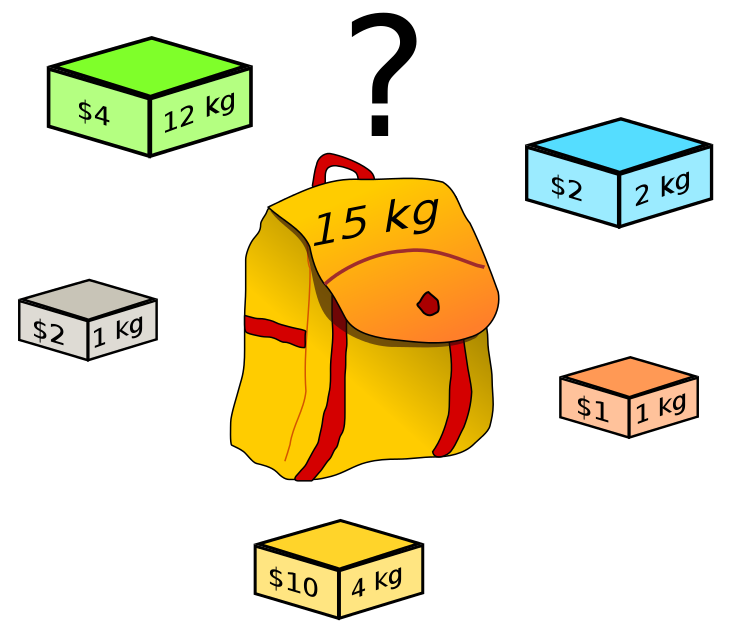Link to the problem page in wiki

Here's the general way the problem is explained - Consider a thief gets into a home to rob and he carries a knapsack. There are fixed number of items in the home - each with its own weight and value - Jewellery, with less weight and highest value vs tables, with less value but a lot heavy. To add fuel to the fire, the thief has an old knapsack which has limited capacity. Obviously, he can't split the table into half or jewellery into 3/4ths. He either takes it or leaves it.

Example :

``````	Knapsack Max weight 	: 		W = 10 (units)

Total items 			: 		N = 4

Values of items 		: 		v[] = {10, 40, 30, 50}

Weight of items 		: 		w[] = {5, 4, 6, 3}
``````

A cursory look at the example data tells us that the max value that we could accommodate with the limit of max weight of 10 is 50 + 40 = 90 with a weight of 7.

## Approach :

The way this is optimally solved is using dynamic programming - solving for smaller sets of knapsack problems and then expanding them for the bigger problem.

Let's build an Item x Weight array called V (Value array)

``````	V[N][W] = 4 rows * 10 columns
``````

Each of the values in this matrix represent a smaller Knapsack problem.

Base case 1 : Let's take the case of 0th column. It just means that the knapsack has 0 capacity. What can you hold in them? Nothing. So, let's fill them up all with 0s

Base case 2 : Let's take the case of 0 row. It just means that there are no items in the house. What do you do hold in your knapsack if there are no items. Nothing again !!! All zeroes.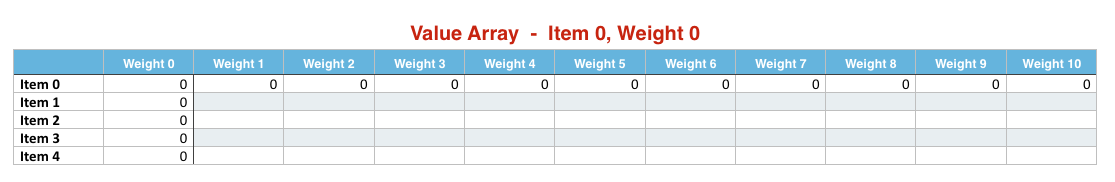## Solution :

1. Now, let's start filling in the array row-wise. What does row 1 and column 1 mean? That given the first item (row), can you accommodate it in the knapsack with capacity 1 (column). Nope. The weight of the first item is 5. So, let's fill in 0. In fact, we wouldn't be able to fill in anything until we reach the column 5 (weight 5).

2. Once we reach column 5 (which represents weight 5) on the first row, it means that we could accommodate item 1. Let's fill in 10 there (remember, this is a Value array)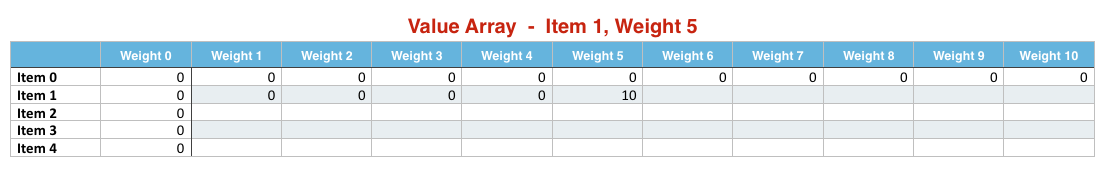1. Moving on, for weight 6 (column 6), can we accommodate anything else with the remaining weight of 1 (weight - weight of this item => 6 - 5). Hey, remember, we are on the first item. So, it is kind of intuitive that the rest of the row will just be the same value too since we are unable to add in any other item for that extra weight that we have got.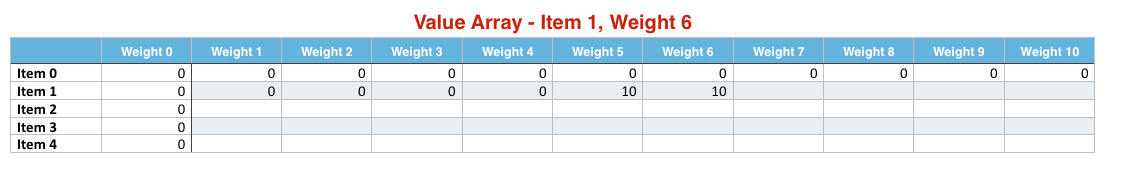1. So, the next interesting thing happens when we reach the column 4 in third row. The current running weight is 4.

We should check for the following cases.

1. Can we accommodate Item 2 - Yes, we can. Item 2's weight is 4.
2. Is the value for the current weight is higher without Item 2? - Check the previous row for the same weight. Nope. the previous row* has 0 in it, since we were not able able accommodate Item 1 in weight 4.
3. Can we accommodate two items in the same weight so that we could maximize the value? - Nope. The remaining weight after deducting the Item2's weight is 0.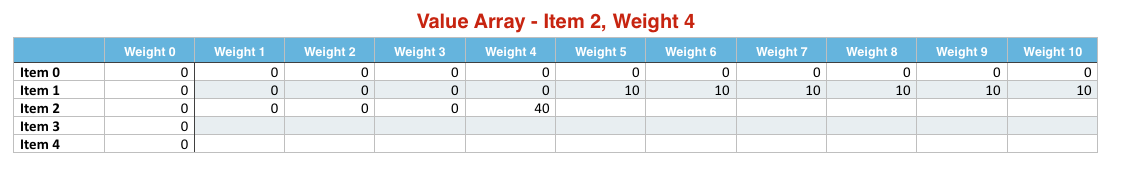### Why previous row ?

Simply because the previous row at weight 4 itself is a smaller knapsack solution which gives the max value that could be accumulated for that weight until that point (traversing through the items).

Exemplifying,

1. The value of the current item = 40
2. The weight of the current item = 4
3. The weight that is left over = 4 - 4 = 0
4. Check the row above (the Item above in case of Item 1 or the cumulative Max value in case of the rest of the rows). For the remaining weight 0, are we able to accommodate Item 1? Simply put, is there any value at all in the row above for the given weight)

The calculation goes like so :

1. Take the max value for the same weight without this item

previous row, same weight = 0

=> V[item-1][weight]

2. Take the value of the current item + value that we could accommodate with the remaining weight

Value of current item

• value in previous row with weight 4 (total weight until now (4) - weight of the current item (4))

=> val[item-1] + V[item-1][weight-wt[item-1]]

Max among the two is 40 (0 and 40)

1. The next and the most important event happens at column 9 and row 2. Meaning we have a weight of 9 and we have two items. Looking at the example data we could accommodate the first two items. Here, we consider few things

1. The value of the current item = 40
2. The weight of the current item = 4
3. The weight that is left over = 9 - 4 = 5
4. Check the row above. At the remaining weight 5, are we able to accommodate Item 1.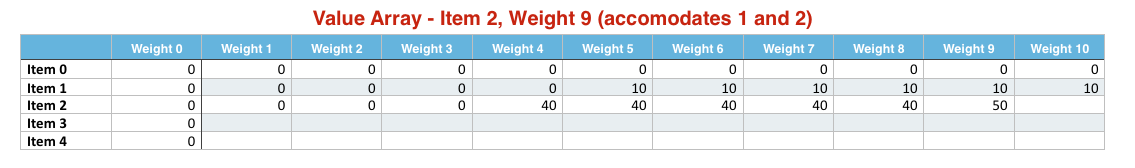So, the calculation is :

1. Take the max value for the same weight without this item

previous row, same weight = 10

2. Take the value of the current item + value that we could accumulate with the remaining weight

Value of current item (40)

• value in previous row with weight 5 (total weight until now (9) - weight of the current item (4))

= 10

10 vs 50 = 50

At the end of solving all these smaller problems, we just need to return the value at V[N][W] - Item 4 at Weight 10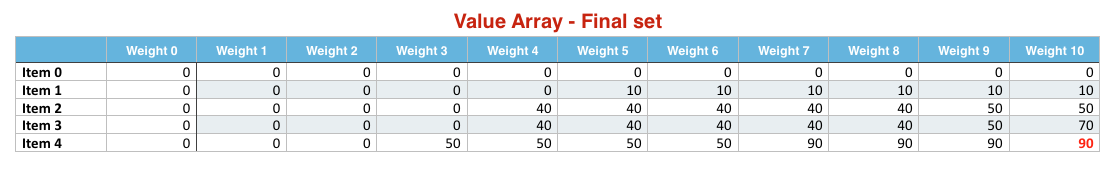## Complexity

Analyzing the complexity of the solution is pretty straight-forward. We just have a loop for W within a loop of N => O (NW)

## Implementation :

Here comes the obligatory implementation code in Java

``````
class Knapsack {

public static void main(String[] args) throws Exception {
int val[] = {10, 40, 30, 50};
int wt[] = {5, 4, 6, 3};
int W = 10;

System.out.println(knapsack(val, wt, W));
}

public static int knapsack(int val[], int wt[], int W) {

//Get the total number of items.
//Could be wt.length or val.length. Doesn't matter
int N = wt.length;

//Create a matrix.
//Items are in rows and weight at in columns +1 on each side
int[][] V = new int[N + 1][W + 1];

//What if the knapsack's capacity is 0 - Set
//all columns at row 0 to be 0
for (int col = 0; col <= W; col++) {
V[col] = 0;
}

//What if there are no items at home.
//Fill the first row with 0
for (int row = 0; row <= N; row++) {
V[row] = 0;
}

for (int item=1;item<=N;item++){

//Let's fill the values row by row
for (int weight=1;weight<=W;weight++){

//Is the current items weight less
//than or equal to running weight
if (wt[item-1]<=weight){

//Given a weight, check if the value of the current
//item + value of the item that we could afford
//with the remaining weight is greater than the value
//without the current item itself
V[item][weight]=Math.max (val[item-1]+V[item-1][weight-wt[item-1]], V[item-1][weight]);
}
else {
//If the current item's weight is more than the
//running weight, just carry forward the value
//without the current item
V[item][weight]=V[item-1][weight];
}
}

}

//Printing the matrix
for (int[] rows : V) {
for (int col : rows) {

System.out.format("%5d", col);
}
System.out.println();
}

return V[N][W];

}

}

``````